# OOF2: The Manual

## Name

Rodrigues (Rodrigues) — Rodrigues vector representation for 3D orientations. The vector points along the axis of the rotation, and its magnitude is the tangent of half the angle of the rotation. The rotation brings the crystal axes into coincidence with the lab axes.

## Synopsis

Rodrigues(r1,r2,r3)

## Details

• Base class: Orientation
• Parameters:

r1
x component of Rodrigues vector. Type: A real number.
r2
y component of Rodrigues vector. Type: A real number.
r3
z component of Rodrigues vector. Type: A real number.

## Description

A Rodrigues object represents the orientation of a three dimensional object, assumed to be a crystal, in three dimensional space in terms of three parameters, representing the axis around which the rotation occurs, and the angle of the rotation. This scheme resembles the Axis scheme, except that there is no explicit angle parameter, the angle being encoded in the magnitude of the vector.

The physical rotation generated by the parameters brings the crystalline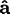,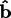, and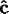axes into coincidence with the laboratory (or screen) axes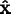,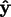, and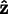respectively. The rotation occurs around a line joining the origin to the point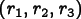, and the angle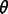of the rotation is given by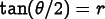, with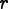being the magnitude of the vector.